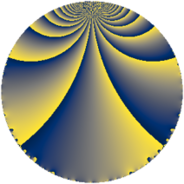# Properties

 Label 120.2.kLevel $120$ Weight $2$ Character orbit 120.k Rep. character $\chi_{120}(61,\cdot)$ Character field $\Q$ Dimension $8$ Newform subspaces $2$ Sturm bound $48$ Trace bound $1$

# Related objects

## Defining parameters

 Level: $$N$$ $$=$$ $$120 = 2^{3} \cdot 3 \cdot 5$$ Weight: $$k$$ $$=$$ $$2$$ Character orbit: $$[\chi]$$ $$=$$ 120.k (of order $$2$$ and degree $$1$$) Character conductor: $$\operatorname{cond}(\chi)$$ $$=$$ $$8$$ Character field: $$\Q$$ Newform subspaces: $$2$$ Sturm bound: $$48$$ Trace bound: $$1$$ Distinguishing $$T_p$$: $$7$$

## Dimensions

The following table gives the dimensions of various subspaces of $$M_{2}(120, [\chi])$$.

Total New Old
Modular forms 28 8 20
Cusp forms 20 8 12
Eisenstein series 8 0 8

## Trace form

 $$8q + 4q^{2} - 2q^{4} + 2q^{6} + 8q^{7} + 4q^{8} - 8q^{9} + O(q^{10})$$ $$8q + 4q^{2} - 2q^{4} + 2q^{6} + 8q^{7} + 4q^{8} - 8q^{9} - 2q^{10} - 12q^{14} - 4q^{15} + 2q^{16} - 4q^{18} - 8q^{20} - 12q^{22} - 16q^{23} + 10q^{24} - 8q^{25} + 28q^{26} - 28q^{28} + 8q^{31} + 4q^{32} + 2q^{36} + 2q^{40} + 12q^{42} + 12q^{44} - 28q^{46} + 16q^{48} + 24q^{49} - 4q^{50} - 8q^{52} - 2q^{54} + 16q^{55} - 4q^{56} - 16q^{57} - 12q^{58} + 2q^{60} + 24q^{62} - 8q^{63} + 22q^{64} + 4q^{66} - 16q^{68} + 4q^{70} - 16q^{71} - 4q^{72} - 16q^{73} + 20q^{74} + 28q^{76} + 8q^{78} + 8q^{79} + 16q^{80} + 8q^{81} + 48q^{82} - 12q^{84} - 24q^{86} + 24q^{87} + 28q^{88} + 2q^{90} - 24q^{92} - 4q^{94} - 18q^{96} + 16q^{97} - 12q^{98} + O(q^{100})$$

## Decomposition of $$S_{2}^{\mathrm{new}}(120, [\chi])$$ into newform subspaces

Label Dim. $$A$$ Field CM Traces $q$-expansion
$$a_2$$ $$a_3$$ $$a_5$$ $$a_7$$
120.2.k.a $$2$$ $$0.958$$ $$\Q(\sqrt{-1})$$ None $$2$$ $$0$$ $$0$$ $$4$$ $$q+(1+i)q^{2}-iq^{3}+2iq^{4}+iq^{5}+\cdots$$
120.2.k.b $$6$$ $$0.958$$ 6.0.399424.1 None $$2$$ $$0$$ $$0$$ $$4$$ $$q+\beta _{2}q^{2}+\beta _{1}q^{3}+(\beta _{1}+\beta _{5})q^{4}+\beta _{1}q^{5}+\cdots$$

## Decomposition of $$S_{2}^{\mathrm{old}}(120, [\chi])$$ into lower level spaces

$$S_{2}^{\mathrm{old}}(120, [\chi]) \cong$$ $$S_{2}^{\mathrm{new}}(24, [\chi])$$$$^{\oplus 2}$$$$\oplus$$$$S_{2}^{\mathrm{new}}(40, [\chi])$$$$^{\oplus 2}$$